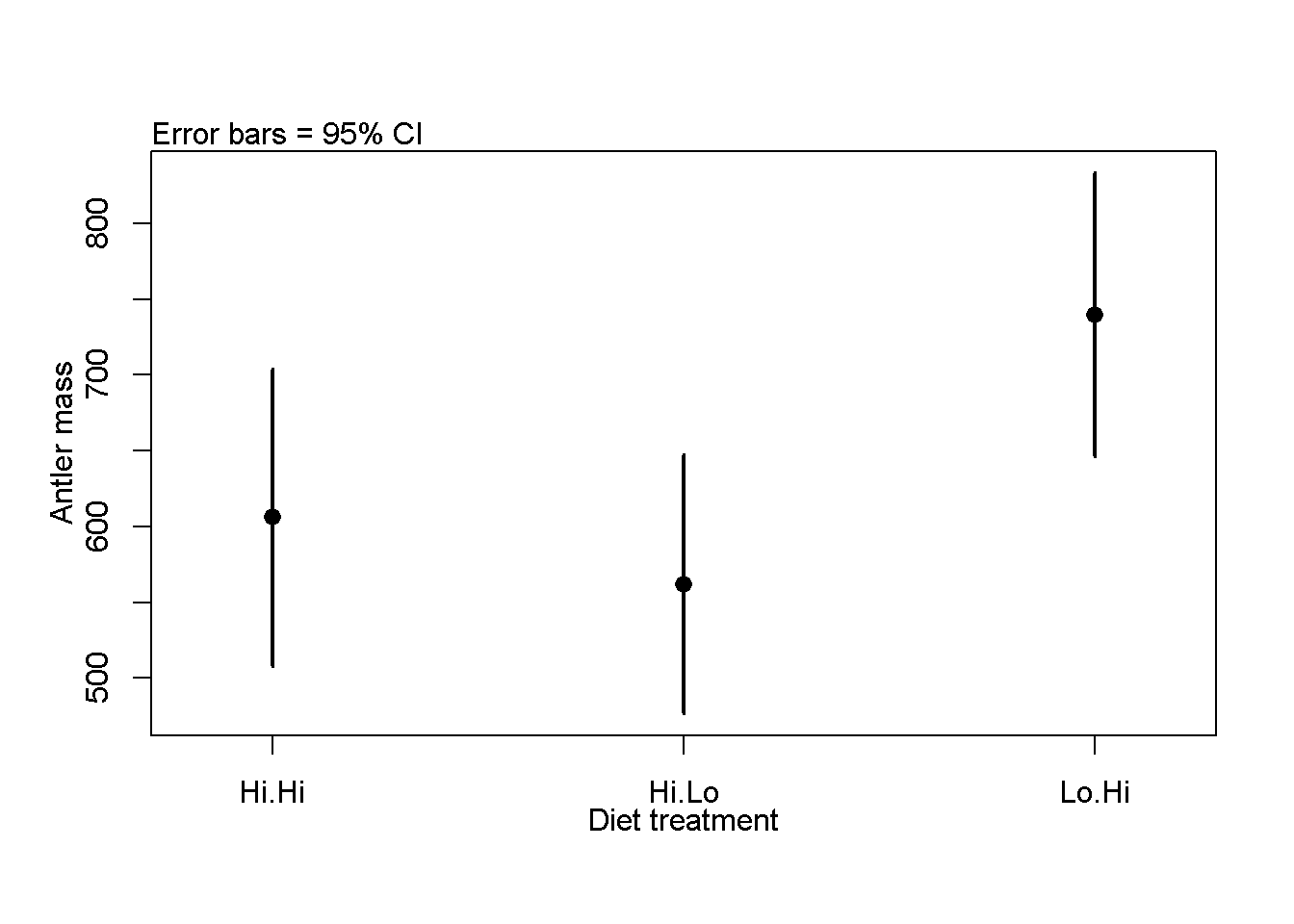1 Inspiration

Asleson et al. 1997. Effects of seasonal protein restriction on antlerogenesis and body mass in adult male white-tailed deer. Journal of Wildlife Management 61.

2 Create data

Make dataframe for analysis

mass<-c(472.5114,722.7774,548.6796,464.3168,577.2037,406.7964,555.8052,657.5226,734.7416,919.2983,665.6099,570.3627,429.5572,520.9726,346.0116,639.3096,544.8374,657.6512,802.2045,443.339,545.9901,791.3983,884.5803,763.2926,782.1005,840.2083,417.7051,735.8959,759.7498,877.0629)

n <- length(mass)/3

diet <- c(rep("Hi.Hi",n),rep("Hi.Lo",n),rep("Lo.Hi",n))

antlers <- data.frame(antler.mass = mass,
diet = diet)

rm(list = c("mass", "diet","n"))

Look at raw antler data

summary(antlers)
##   antler.mass       diet
##  Min.   :346.0   Hi.Hi:10
##  1st Qu.:526.9   Hi.Lo:10
##  Median :648.4   Lo.Hi:10
##  Mean   :635.9
##  3rd Qu.:762.4
##  Max.   :919.3

3 plot3means function

Need to load this function (or more general plotmeans() )

4 Part 1: Summary stats & plotting means

4.1 1) Summary stats on all data

In this section the grand mean on ALL the data is calculated; data is NOT broken up by treatment! Data is considered by subgroup below

# total sample size (all observations)
dim(antlers)
##  30  2
n.total <- length(antlers\$antler.mass)

#mean of ALL samples
summary(antlers)
##   antler.mass       diet
##  Min.   :346.0   Hi.Hi:10
##  1st Qu.:526.9   Hi.Lo:10
##  Median :648.4   Lo.Hi:10
##  Mean   :635.9
##  3rd Qu.:762.4
##  Max.   :919.3
mean(antlers\$antler.mass)
##  635.9164
#variance of ALL samples
var(antlers\$antler.mass)
##  25767.67
#stdev of ALL samples
mass.sd <- sd(antlers\$antler.mass)

4.2 2) SE for ** all ** data

This ignores treatments. All data are combined / pooled.

#Using saved sd
mass.se <- mass.sd/sqrt(n.total)

sqrt.n <- sqrt(n.total)

mass.se <- mass.sd/sqrt.n

#Using raw data
mass.se <- sd(antlers\$antler.mass)/
sqrt(length(antlers\$antler.mass))

4.3 3) 95% CI for all data

This is the 95% confidence interval around the overall/grand mean.

1.96*mass.se
##  57.44246

4.4 4) Calcualte summary stats for each group

Each group is considered seperately.

# Means
mass.3.means <- tapply(antlers\$antler.mass,
antlers\$diet,
mean)

#SD
mass.3.sd <- tapply(antlers\$antler.mass,
antlers\$diet,
sd)

#sample size n
mass.3.n <- tapply(antlers\$antler.mass,
antlers\$diet,
length)

4.5 5) Calcualte the 3 SE values

mass.3.se <- mass.3.sd/sqrt(mass.3.n)

4.6 6) Plot the 3 mean valeus

#Using the levels command
antler.groups <- levels(antlers\$diet)

#By hand
antler.groups <- c("Hi.Hi", "Hi.Lo", "Lo.Hi")

plot3means(means = mass.3.means,
se = mass.3.se,
categories = antler.groups,
x.axis.label = "Diet treatment",
y.axis.label = "Antler mass"
)##  "This function plots approximate 95% confidence intervals based on standard error imput by the user.  DO not enter 95% CIs"
boxplot(antler.mass ~ diet, data = antlers)